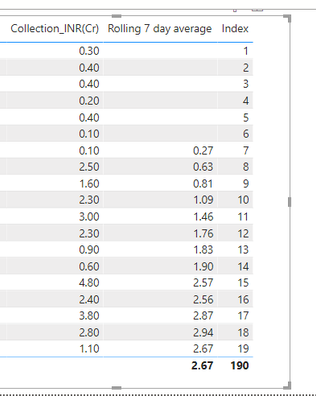cancel
Showing results for
Did you mean:Resolver I

## How to create a measure to calculated Rolling 7 day average to use in matrix ?

Hi All,

I have a day-wise collection matrix visual and in the next field of the matrix visual,  I want to show the Rolling 7-day average collection.

Refer to the below sample table ( in power bi this is matrix visual) where I have type desired output. I need output like this.

 Day Collection_INR(Cr) Rolling 7 day average Coll (desired output) 01-Nov 0.3 - 02-Nov 0.4 - 03-Nov 0.4 - 04-Nov 0.2 - 05-Nov 0.4 - 06-Nov 0.1 - 07-Nov 0.1 0.3 08-Nov 2.5 0.6 09-Nov 1.6 0.8 10-Nov 2.3 1.0 11-Nov 3 1.4 12-Nov 2.3 1.7 13-Nov 0.9 1.8 14-Nov 0.6 1.9 15-Nov 4.8 2.2 16-Nov 2.4 2.3 17-Nov 3.8 2.5 18-Nov 2.8 2.5 19-Nov 1.1 2.3

Thanks

Lavdeep

1 ACCEPTED SOLUTIONCommunity Support

Hi @lavdeepk ,

With the index column created, try the following code.

``````Rolling 7 day average =
IF (
( MAX ( 'Table'[Index] ) - 7 ) < 0,
BLANK (),
CALCULATE (
SUM ( 'Table'[Collection_INR(Cr)] ),
FILTER (
ALL ( 'Table' ),
'Table'[Index] <= MAX ( 'Table'[Index] )
&& 'Table'[Index]
>= MAX ( 'Table'[Index] ) - 7
)
) / 7
)``````Best Regards,
Henry

If this post helps, then please consider Accept it as the solution to help the other members find it more quickly.

2 REPLIES 2Community Support

Hi @lavdeepk ,

With the index column created, try the following code.

``````Rolling 7 day average =
IF (
( MAX ( 'Table'[Index] ) - 7 ) < 0,
BLANK (),
CALCULATE (
SUM ( 'Table'[Collection_INR(Cr)] ),
FILTER (
ALL ( 'Table' ),
'Table'[Index] <= MAX ( 'Table'[Index] )
&& 'Table'[Index]
>= MAX ( 'Table'[Index] ) - 7
)
) / 7
)``````Best Regards,
Henry

If this post helps, then please consider Accept it as the solution to help the other members find it more quickly.Super User

Try:

``````Rolling 7 day average =
AVERAGEX (
FILTER (
ALL ( 'Date Table'[Date] ),
'Date Table'[Date]
> MAX ( 'Date Table'[Date] ) - 7
&& 'Date Table'[Date] <= MAX ( 'Date Table'[Date] )
),
SUM ( 'Table'[Collection INR] )
)
``````

In doing so, you are also helping me. Thank you!

Proud to be a Super User!